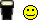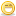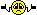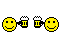Thread: OLFA table vs. Custom OS A0008 table

1.OLFA table vs. Custom OS A0008 table

My custom OS does not have OLFA, it has a A0008 file. The A0008 states its a multiplier. Is this truely a multiplier or is it a divider?

Thanks,
GlennReply With Quote

2.It is a multiplier. I'm going to assume that your display units are AFR or EQ Ratio , and that the commanded AFR is 14.63:1 (or EQ Ratio of 1.0) for the example.

- A multiplier of 1.00 the commanded AFR will be 14.63:1
- A multiplier of 1.20 the commanded AFR will be 12.19:1 (Richer)
- A multiplier of 0.80 the commanded AFR will be 18.29:1 (Leaner)

The multiplier is for enrichment (richening the mixture) and the lower the AFR numbers are richer the mixture.

Hope that makes sense.Reply With Quote

3.how is 0.80*14.629=18.29 a multiplication?i'd call it a divisionReply With Quote

4.Originally Posted by redhardsupra
how is 0.80*14.629=18.29 a multiplication?i'd call it a division
No wonder my AFRs are out of whackReply With Quote

5.All I can say is 'whatever', a higher number is more fuel. Multipliers generally give 'more', which in this case is fuel.

Either way, that's how it works.Reply With Quote

6.Originally Posted by redhardsupra
how is 0.80*14.629=18.29 a multiplication?i'd call it a division
If you look at it on EQ basis, it's a multiplierReply With Quote

7.Could have sworn Lamda was the multiplier and EQ was the divisor.Reply With Quote

8.Originally Posted by Garry
If you look at it on EQ basis, it's a multiplierThis is the key.It multiplies the current EQ to get the target EQ; the current EQ equals 14.63 divided by current AFR.

AFR = 14.63 / EQ = 14.63 * Lambda

or

EQ = 14.63 / AFR = 1 / Lambda

EQ, Lambda, AFR are all units of how much air/fuel.

A008 is a multipler in the sense that it multiplies current EQ to give target EQ.

Like Tordne said, the higher numbers are richer.

Edit: the more I write here, the muddier it is becoming...Reply With Quote

9.Okay, I am going to try what Tordne said and see what happens.

The whole multiplier/division thing confuses me; however, I understand what is being stated!

I will let you know how it goes.

Thanks for all of the help!

GLennReply With Quote

10.Originally Posted by redhardsupra
how is 0.80*14.629=18.29 a multiplication?i'd call it a division
Thats what i am trying to wrap my head around...lol. My commanded AFR is 14.70:1 and my multiplier (for a specific cell) is 1.0229. 14.70 x 1.0229 = 15.036 which equals a leaner AFR. So regardless of the table description of "multiplier", if i put a number larger than 1.00 in there, thats going to equal more fuel right?Reply With QuotePosting Permissions

• You may not post new threads
• You may not post replies
• You may not post attachments
• You may not edit your posts
•